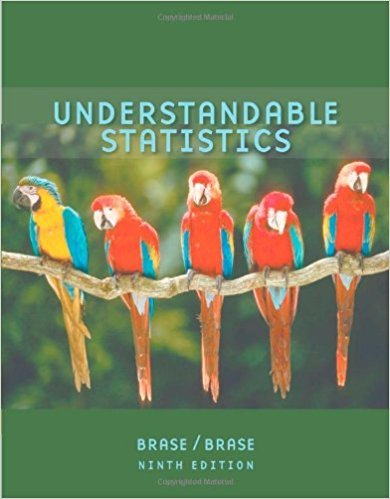×
Get Full Access to Understandable Statistics - 9 Edition - Chapter 9.1 - Problem 1
Get Full Access to Understandable Statistics - 9 Edition - Chapter 9.1 - Problem 1

×

# Statistical Literacy Discuss each of the following topicsISBN: 9780618949922 213

## Solution for problem 1 Chapter 9.1

Understandable Statistics | 9th Edition

• Textbook Solutions
• 2901 Step-by-step solutions solved by professors and subject experts
• Get 24/7 help from StudySoup virtual teaching assistantsUnderstandable Statistics | 9th Edition

4 5 1 430 Reviews
21
1
Problem 1

Statistical Literacy Discuss each of the following topics in class or review the topics on your own. Then write a brief but complete essay in which you answer the following questions. (a) What is a null hypothesis H0? (b) What is an alternate hypothesis H1? (c) What is a type I error? a type II error? (d) What is the level of significance of a test? What is the probability of a type II error?

Step-by-Step Solution:
Step 1 of 3

Statistics 401 Notes Statistics is about collecting data, organizing it, summarization, and then analyzing it. Stats  Collecting o Ex: Surveys  Organization o Grouping the data using graphs or charts  Summarization o Finding the average- median, mean, mode o (center and spread of data- Ex: Standard deviation) o The lesser spread of data (smaller range) = a more reliable source because it is more consistent.  Ex: Data with outcomes of 30-70 (40 range) is MORE reliable than a range from 0-100 (100 range) o Population is the set of all units under study  Population is represented by a sample of these units (random sample).

Step 2 of 3

Step 3 of 3

##### ISBN: 9780618949922

Unlock Textbook Solution